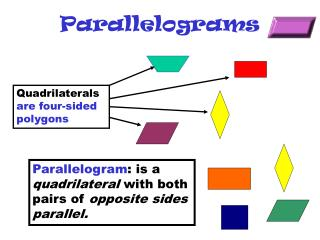DownloadDownload PresentationParallelograms

# Parallelograms

Download Presentation## Parallelograms

- - - - - - - - - - - - - - - - - - - - - - - - - - - E N D - - - - - - - - - - - - - - - - - - - - - - - - - - -
##### Presentation Transcript

1. Parallelograms Quadrilaterals are four-sided polygons Parallelogram: is a quadrilateral with both pairs of opposite sides parallel.

2. A B Parallelograms (2) D C Theorem 6.1 : Opposite sides of a parallelograms are congruent AD  BC and AB  DC Theorem 6.2: Opposite angles of a parallelogram are congruent <A  <C and <B  <D m<A+m<B = 180° m <B+m<C = 180° m<C+m<D = 180° m<D+m<A = 180° Theorem 6.3: Consecutive angles in a parallelogram are supplementary.

3. Parallelograms (3) Diagonals of a figure: Segments that connect any to vertices of a polygon A B Theorem 6.4: The diagonals of a parallelogram bisect each other. D C

4. Parallelograms (4) • Draw a parallelogram : ABCD on a piece of construction paper. • Cut the parallelogram. • Fold the paper and make a crease from A to C and from B to D. • Fold the paper so A lies on C. What do you observe? • Fold the paper so B lies on D. What do you observe? • What theorem is confirmed by these Observations?

5. Tests for Parallelograms Theorem 6.5 :If both pairs of opposite sides of a quadrilateral are congruent, then the quadrilateral is a parallelogram. A If AD  BC and AB  DC, then ABCD is a parallelogram B D C Theorem 6.6:If both pairs of opposite angles of a quadrilateral are congruent, then the quadrilateral is a parallelogram. If <A  <C and <B  <D, then ABCD is a parallelogram

6. Tests for Parallelograms 2 Theorem 6.7: If the diagonals of a quadrilateral bisect each other, then the quadrilateral is a parallelogram A B D C Theorem 6.8: If one pair of opposite sides of a quadrilateral is both parallel and congruent, then the quadrilateral is a parallelogram.

7. A quadrilateral is a parallelogram if... Both pairs of opposite sides are parallel.(Definition) Both pairs of opposite sides are congruent.(Theorem 6.5) Both pairs of opposite angles are congruent. (Theorem 6.6) Diagonals bisect each other. (Theorem 6.7) A pair of opposite sides is both parallel and congruent. (Theorem 6.8)

8. Area of a parallelogram If a parallelogram has an area of A square units, a base of b units and a height of h units, then A = bh. (Do example 1 p. 530) h b The area of a region is the sum of the areas of all its non-overlapping parts. (Do example 3 p. 531)

9. Rectangles A rectangle is a quadrilateral with four right angles. Opp. angles in rectangles are congruent (they are right angles) thereforerectangles are parallelograms with all their properties. Theorem 6-9 : If a parallelogram is a rectangle, then its diagonals are congruent. Theorem 6-10 : If the diagonals of a parallelogrma are congruent then the parallelogram is a rectangle.

10. Rectangles (2) • If a quadrilateral is a rectangle, then the following properties hold true: • Opp. Sides are congruent and parallel • Opp. Angles are congruent • Consecutive angles are supplementary • Diagonals are congruent and bisect each other • All four angles are right angles

11. Squares and Rhombi A rhombus is a quadrilateral with four congruent sides. Since opp. sides are ,a rhombus is a parallelogram with all its properties. Special facts about rhombi Theorem 6.11: The diagonals of a rhombus are perpendicular. Theorem 6.12: If the diagonals of a parallelogram are perpendicular, then the parallelogram is a rhombus. Theorem 6.13: Each diagonal of a rhombus bisects a pair of opp. angles C

12. Squares and Rhombi(2) If a rhombus has an area of A square units and diagonals of d1 and d2 units, then A = ½ d1d2. If a quadrilateral is both, a rhombus and a rectangle, is asquare

13. Area of a triangle: h b If a triangle has an area of A square units a base of b units and corresponding height of h units, then A = ½bh. Congruent figures have equal areas.

14. Trapezoids A trapezoid is a quadrilateral with exactly one pair of parallel sides. The parallel sides are called bases. The nonparallel sides are called legs. At each side of a base there is a pair of base angles. C

15. Trapezoids (2) A B AB = base CD = base AC = leg BD = leg AB  CD AC & BD are non parallel C D <A & <B = pair of base angles <C & <D = pair of base angles C

16. Trapezoids (3) Isosceles trapezoid: A trapezoid with congruent legs. Theorem 6-14: Both pairs of base angles of an isosceles trapezoid are congruent. Theorem 6-15: The diagonals of an isosceles trapezoid are congruent.

17. Trapezoids (4) The median of a trapezoid is the segment that joints the midpoints of the legs (PQ). A B P Q C D Theorem 6-16: The median of a trapezoid is parallel to the bases, and its measure is one-half the sum of the measures of its bases. C

18. Area of Trapezoids B A h C D Area of a trapezoid:If a trapezoid has an area of A square units, bases of b1 and b2 units and height of h units, then A = ½(b1 + b2 )h. C

19. This powerpoint was kindly donated to www.worldofteaching.com http://www.worldofteaching.com is home to over a thousand powerpoints submitted by teachers. This is a completely free site and requires no registration. Please visit and I hope it will help in your teaching.